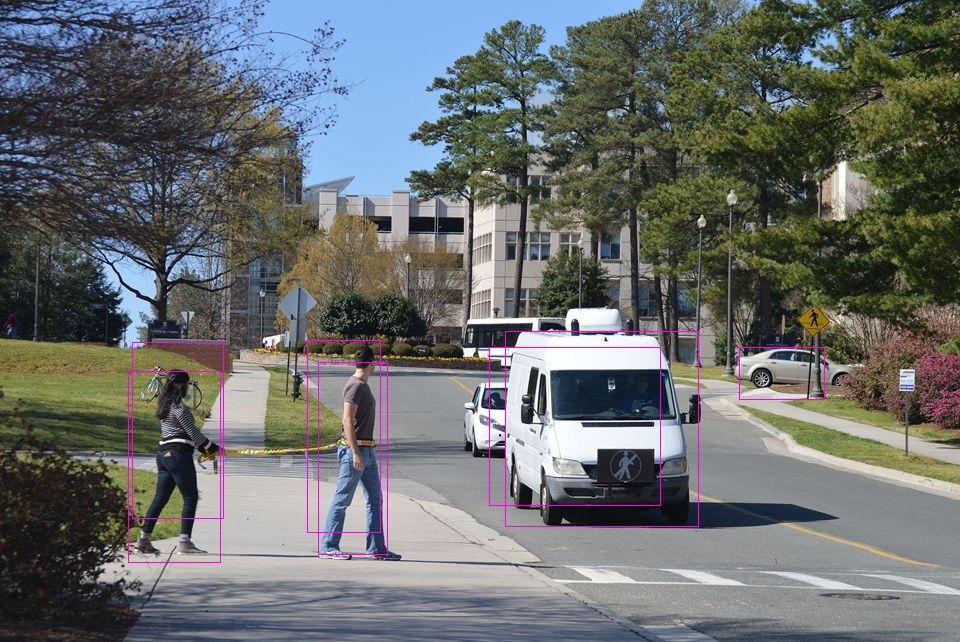#### SOD C/C++ API Reference - Image Processing

Syntax

``void sod_image_draw_box(sod_img input, int x1, int y1, int x2, int y2, float r, float g, float b);``

Description

Draw a single box (i.e. rectangle) on an input image. As of this release, we recommend using sod_image_draw_bbox() instead. This function is very useful when working with the CNN/Realnet interfaces for example to mark a detected object (i.e. car, plane, person, etc.) coordinates. When done, you can save the output on disk via sod_img_save_as_png() for instance.

Typical OutputParameters

sod_img    input

The input image to be processed. The image can be loaded from disk using sod_img_load_from_file(), from memory (i.e. network socket) via sod_img_load_from_mem() or dynamically created via sod_make_image().

int    x1

The x-coordinate, in logical units of the rectangle starting position.

int    y1

The y-coordinate, in logical units of the rectangle starting position.

int    x2

The x-coordinate, in logical units of the rectangle ending position. So x2 - x1 should give you the width of this rectangle.

int    y2

The y-coordinate, in logical units of the rectangle ending position. So y2 - y1 should give you the height of this rectangle.

float    r

Red color value in a typical RGB colorspace. This interface handle grayscale colorspace also.

float    g

Green color value in a typical RGB colorspace.

float    b

Blue color value in a typical RGB colorspace.

Return Value

None.

Example

Checkout the introduction course, the C/C++ samples on the download page or refer to the SOD Github Repository.